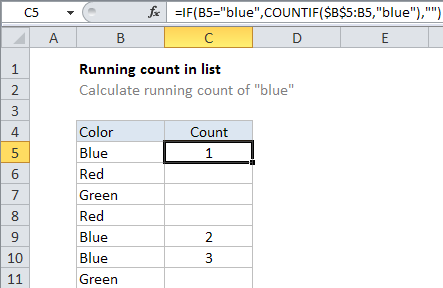## Excel Office

Excel How Tos, Tutorials, Tips & Tricks, Shortcuts

# Running count of occurrence in list in Excel

This tutorial shows how to work Running count of occurrence in list in Excel using the example below;

## Formula

```=COUNTIF(\$A\$1:A1,value)```

## Explanation

To create a running count of certain values that appear in range of cells, you can use the COUNTIF with a “mixed reference” to create a running total. In the example shown, the formula in C5 is:

`=IF(B5="blue",COUNTIF(\$B\$5:B5,"blue"),"")`

### How this formula works

Working from the inside out, the  COUNTIF function is set up to count the value “blue” that appears in column B:

`COUNTIF(\$B\$5:B5,"blue")`

Note the left side of the range reference is locked (\$B\$5) and the right side is relative (B5).  This is called a “mixed reference”, since it contains both absolute and relative addresses, and it creates an expanding range.

Also See:   Basic text sort formula in Excel

As the formula is copied, the first cell in the reference is locked, but the second reference expands to include each new row. On each row, COUNTIF counts cells in the range that are equal to “blue”, and the result is a running count.

The outer layer of the formula uses the IF function to control when COUNTIF fires. COUNTIF only generated a count when the value in B is “blue”. If not, IF returns an empty string (“”).

Also See:   Excel Data validation require unique number

### Running count of every value

To create a running count of every value that appears in column A, you can use a generic version of the formula like this:

`=COUNTIF(\$A\$1:A1,A1)`